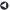### Statistical Services OfferedBack to List of Services

#### Survival Analysis

Survival Analysis is a class of statistical methods used to analyze data in which time until an event occurs is the response variable of interest. In survival analysis, the response variable is often called a failure time, survival time, or event time and is usually continuous, being measured in days, weeks, months, years, etc. Examples of events are deaths, onset of disease, marriages, arrests, etc. A distinguishing feature of survival analysis is that even if the subject did not experience an event, the subject's survival time or length of time in the study is still taken into account.

Clinical and epidemiological follow-up studies employ survival analysis extensively. Other fields which use survival analysis methods include sociology, engineering, and economics. The common objective of survival analysis in these various fields is not just whether an event occurred, but when it occurred.

As noted above, survival analysis can handle cases when the response variable is incompletely determined. These observations are called censored observations and can occur when a subject does not experience the event before the study ends, the subject is lost to follow-up during the study, or the subject withdraws from the study. Censored observations cannot be ignored because longer-lived subjects are generally more likely to be censored.

Survival analysis methods can handle two common features of survival analysis data: censoring and time-dependent explanatory variables. In contrast, neither linear regression nor logistic regression is appropriate for handling survival analysis data. Linear regression cannot handle censored observations nor time-dependent covariates nor the unusual distributions which time-to-event can have. Logistic regression ignores information on the timing of events and cannot handle time-dependent covariates.

Analysis of survival data typically begins with graphing the survival function and comparing functions for sub-groups of the data. The survival function gives the probability that a subject survives longer than some specified time t. A related function is the hazard function which is the instantaneous risk or potential that an event will occur at time t, given that the subject has survived up to time.

The Kaplan-Meier method and the Life Table method are the two main methods for exploring survival functions: The former is usually much more detailed than the latter since its time interval boundaries are determined by the actual event times. The Life Table method (also known as the actuarial method) is useful when the number of event times is large because they can be grouped into intervals. In the Kaplan-Meier method, censored observations are assumed to be at risk for the whole event time period. In the Life Table method, censored observations are censored at the midpoint of the time interval.

After exploratory data analysis, the next major step is the estimation of the hazard function. When the distribution of survival time is known, parametric models, such as the Weibull model, can be used. In many situations, however, the distribution of survival time is unknown or the hazard function is unspecified, so parametric methods are not suitable. The most popular alternative method is the Cox Proportional Hazards Model, which provides the primary information sought from a survival analysis: hazard ratios and adjusted survival curves, with a minimum of assumptions.

Even though the Cox model does not assume any particular distribution for the hazard function, it does make several assumptions which should be checked after the model has been formulated. One of these is the assumption that the hazard for one group is proportional to the hazard of another group, with the proportionality being constant over time. If this assumption is true, the hazard functions of two groups plot as two parallel lines in a plot of the log of the hazard function over time. If the estimated hazard ratios are not constant over time, then a non-proportional hazards model should be used such as the stratified Cox model or the Cox model with time-dependent variables.